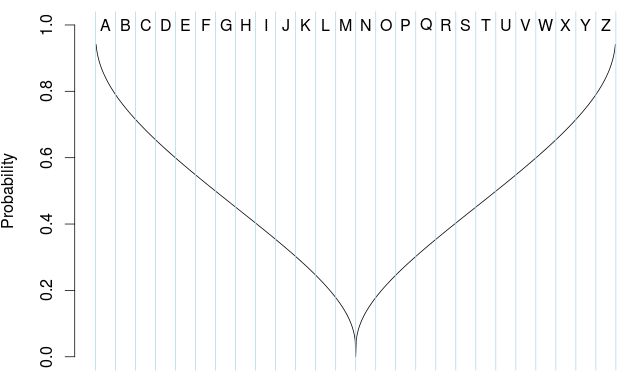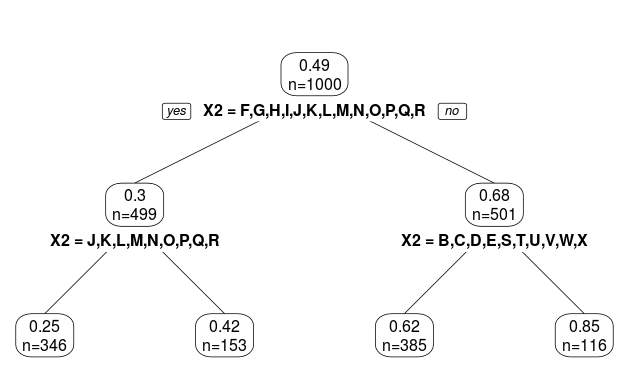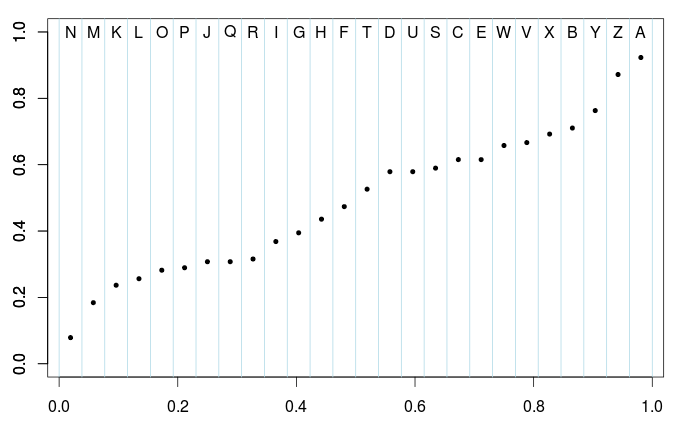Want to share your content on R-bloggers? click here if you have a blog, or here if you don't.

I think that over the past months, I have been saying non-correct things about classification with categorical covariates. Because I never took time to look at it carefuly. Consider some simulated dataset, with a logistic regression,

```> n=1e3
> set.seed(1)
> X1=runif(n)
> q=quantile(X1,(0:26)/26)
> q=0
> X2=cut(X1,q,labels=LETTERS[1:26])
> p=exp(-.1+qnorm(2*(abs(.5-X1))))/(1+exp(-.1+qnorm(2*(abs(.5-X1)))))
> Y=rbinom(n,size=1,p)
> df=data.frame(X1=X1,X2=X2,p=p,Y=Y)```

Here, we use some continuous covariate, except that is considered as not-observed. Instead, we have a categorical covariate with 26 categories. The (theoretical) relationship between the covariate and the probability is given below,

```> vx1=seq(0,1,by=.001)
> vp=exp(-.1+qnorm(2*(abs(.5-vx1))))/(1+exp(-.1+qnorm(2*(abs(.5-vx1)))))
> plot(vx1,vp,type="l")```and the empirical probability, for each modality isIf we run a classification tree, we get

```> library(rpart)
> tree=rpart(Y~X2,data=df)
> library(rpart.plot)
> prp(tree, type=2, extra=1)```To be more specific, the output is here

```> tree
1) root 1000 249.90000 0.4900000
2) X2=F,G,H,I,J,K,L,M,N,O,P,Q,R 499 105.3 0.302
4) X2=J,K,L,M,N,O,P,Q,R 346  65.12 0.25144  *
5) X2=F,G,H,I 153  37.22876 0.4183007       *
3) X2=A,B,C,D,E,S,T,U,V,W,X,Y,Z 501 109.61 0.67
6) X2=B,C,D,E,S,T,U,V,W,X 385  90.38 0.623  *
7) X2=A,Y,Z 116  14.50862 0.8534483         *```

Note that it takes less than a second to get that output. So clearly, we did not look for all combinations between modalities. For the first node, there are like  possible groups, i.e.

`> 67108864`

It is big… not huge, but too big to try all combinations, since that’s only the first node, and we have to do it again on the two leaves, etc. Antoine (aka @ly_antoine) told me – while we were having a coffee after lunch today – the trick to get a fast algorithm, on categories. And as usual, the idea is very clever…

First, we need a function to compute Gini index

```> gini=function(y,classe){
+    T=table(y,classe)
+    nx=apply(T,2,sum)
+    n=sum(T)
+    pxy=T/matrix(rep(nx,each=2),nrow=2)
+    omega=matrix(rep(nx,each=2),nrow=2)/n
+    g=-sum(omega*pxy*(1-pxy))
+    return(g)}```

For the first node, the idea is very simple:

• Compute empirical averages
`> cond_prob=aggregate(df\$Y,by=list(df\$X2),mean)`
• Then sort those values, ,
• Based on that ordering, consider
`> Group_Letters=cond_prob[order(cond_prob\$x),2]`• Then consider (only)  possible partitions,

against

```> v_gini=rep(NA,26)
> for(v in 1:26){
+   CLASSE=df\$X2 %in% Group_Letters[1:v]
+   v_gini[v]=gini(y=df\$Y,classe=CLASSE)
+ }```

If we plot them, we get

`> plot(1:26,v_gini,type="b)`As for continuous variables, we seek for the maximum value, and then, we have our two groups,```> sort(Group_Letters[1:which.max(v_gini)])
 F G H I J K L M N O P Q R```

That’s exactly what we got with the tree function in R,

```1) root 1000 249.90000 0.4900000
2) X2=F,G,H,I,J,K,L,M,N,O,P,Q,R 499 105.30 0.30```

Now, consider the leaf on the left (for instance)

`> sub_df=df[df\$X2 %in% sort(Group_Letters[1:which.max(v_gini)]),]`

Then use the same algorithm as before: sort the conditional means,

```> cond_prob=aggregate(sub_df\$Y,by=
+ list(sub_df\$X2),mean)
> s_Group_Letters=cond_prob[order(cond_prob\$x),2]```

Then compute Gini indices based on groups obtained from that ordering,

```> v_gini=rep(NA,length(sub_Group_Letters))
> for(v in 1:length(sub_Group_Letters)){
+   CLASSE=sub_df\$X2 %in% s_Group_Letters[1:v]
+   v_gini[v]=gini(y=sub_df\$Y,classe=CLASSE)
+ }```

If we plot it, we get our two groups,

```> plot(1:length(s_Group_Letters),v_gini,type="b")
```And the first group is here

```> sort(sub_Group_Letters[1:which.max(v_gini)])
 J K L M N O P Q R```

Again, that’s exactly what we got with the R function

```1) root 1000 249.90000 0.4900000
2) X2=F,G,H,I,J,K,L,M,N,O,P,Q,R 499 105.30 0.30
4) X2=J,K,L,M,N,O,P,Q,R 346  65.12 0.25144  *```

Clever, isn’t?